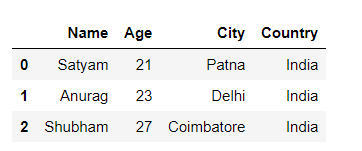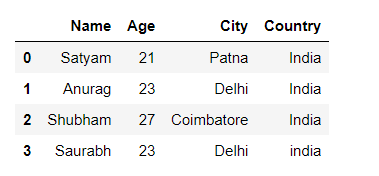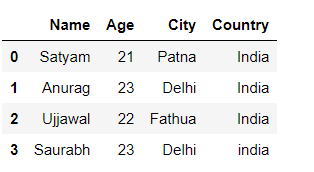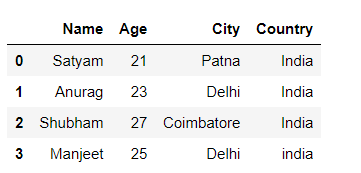# How to append a list as a row to a Pandas DataFrame in Python?

• Last Updated : 05 Oct, 2021

Prerequisite: Pandas DataFrame

In this article, We are going to see how to append a list as a row to a pandas dataframe in Python. It can be done in three ways:

## Append list using loc[] methods

Pandas DataFrame.loc attribute access a group of rows and columns by label(s) or a boolean array in the given DataFrame.

Let’s append the list with step-wise:

Step 1: Create a simple dataframe using the list.

## Python3

 `import` `pandas as pd` `# List``Person ``=` `[ [``'Satyam'``, ``21``, ``'Patna'` `, ``'India'` `],``            ``[``'Anurag'``, ``23``, ``'Delhi'` `, ``'India'` `],``            ``[``'Shubham'``, ``27``, ``'Coimbatore'` `, ``'India'` `]]` `#Create a DataFrame object``df ``=` `pd.DataFrame(Person,``                  ``columns ``=` `[``'Name'` `, ``'Age'``, ``'City'` `, ``'Country'``])` `# display``display(df)`

Output:Step 2: Using loc to append the new list to a data frame.

## Python3

 `# New list for append into df``list` `=` `[``"Saurabh"``, ``23``, ``"Delhi"``, ``"india"``]` `# using loc methods``df.loc[``len``(df)] ``=` `list` `# display``display(df)`

Output:## Append list using iloc[] methods

Pandas DataFrame.iloc method access integer-location based indexing for selection by position.

Example:

## Python3

 `# import module``import` `pandas as pd` `# List``Person ``=` `[ [``'Satyam'``, ``21``, ``'Patna'` `, ``'India'` `],``            ``[``'Anurag'``, ``23``, ``'Delhi'` `, ``'India'` `],``            ``[``'Shubham'``, ``27``, ``'Coimbatore'` `, ``'India'` `],``            ``[``"Saurabh"``, ``23``, ``"Delhi"``, ``"india"``]]` `#Create a DataFrame object``df ``=` `pd.DataFrame(Person,``                  ``columns ``=` `[``'Name'` `, ``'Age'``, ``'City'` `, ``'Country'``])` `# new list to append into df``list` `=` `[``'Ujjawal'``, ``22``, ``'Fathua'``, ``'India'``]` `# using iloc``df.iloc[``2``] ``=` `list` `# display``display(df)`

Output:Note – It is used for location-based indexing so it works for only the existing index and replaces the row element.

## Append list using append() methods

Pandas dataframe.append() function is used to append rows of other dataframe to the end of the given dataframe, returning a new dataframe object.

Example:

## Python3

 `# import module``import` `pandas as pd` `# List``Person ``=` `[ [``'Satyam'``, ``21``, ``'Patna'` `, ``'India'` `],``            ``[``'Anurag'``, ``23``, ``'Delhi'` `, ``'India'` `],``            ``[``'Shubham'``, ``27``, ``'Coimbatore'` `, ``'India'` `]]` `#Create a DataFrame object``df ``=` `pd.DataFrame(Person,``                  ``columns ``=` `[``'Name'` `, ``'Age'``, ``'City'` `, ``'Country'``])` `# new list to append into df``list` `=` `[[``"Manjeet"``, ``25``, ``"Delhi"``, ``"india"``]]` `# using append``df ``=` `df.append(pd.DataFrame( ``list``,``               ``columns``=``[ ``'Name'``, ``'Age'``, ``'City'``, ``'Country'``]),``               ``ignore_index ``=` `True``)` `# display df``display(df)`

Output:My Personal Notes arrow_drop_up